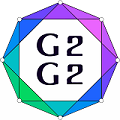#Graphs and Groups, Geometries and GAP (G2G2) Summer School - External Satellite Conference of 8ECM

27 June 2021 to 3 July 2021
Rogla, Slovenia
UTC timezone

## On coincidence of Gruenberg–Kegel graphs of non-isomorphic finite groups

Not scheduled
20m
Rogla, Slovenia

#### Rogla, Slovenia

Hotel Planja, Rogla 1, 3214 Zreče, Slovenia
Oral presentation

Kristina Ilenko

### Description

We use mainly standard notation and terminology (see ). Let $G$ be a finite group. Denote by $\pi(G)$ the set of all prime divisors of the order of $G$ and by $\omega (G)$ the spectrum of $G$, i.e. the set of all its element orders. The set $\omega(G)$ defines the Gruenberg–Kegel graph (or the prime graph) $\Gamma(G)$ of $G$; in this simple graph the vertex set is $\pi(G)$, and distinct vertices $p$ and $q$ are adjacent if and only if $pq\in\omega (G)$.

The problem of description of the cases when Gruenberg–Kegel graphs of non-isomorphic finite groups coincide naturally arises. One of the most interesting cases of this problem is when Gruenberg–Kegel graphs of non-isomorphic finite groups are both disconnected and coincide.

Recall some known definitions. A finite group $G$ is a Frobenius group if there is a non-trivial subgroup $C$ of $G$ such that $C \cap gCg^{-1} = \{1\}$ whenever $g \not \in C$. A subgroup $C$ is a Frobenius complement of $G$. Let $K=\{1\} \cup (G\setminus \bigcup_{g \in G} gCg^{-1}).$ Then $K$ is a normal subgroup of a Frobenius group $G$ with a Frobenius complement $C$ which is called the Frobenius kernel of $G$. A finite group $G$ is a $2$-Frobenius group if $G=ABC$, where $A$ and $AB$ are normal subgroups of $G$, $AB$ and $BC$ are Frobenius groups with kernels $A$ and $B$ and complements $B$ and $C$, respectively. It is known that each $2$-Frobenius group is solvable. The socle $Soc(G)$ of a finite group $G$ is the subgroup of $G$ generated by the set of all its non-trivial minimal normal subgroups. A finite group $G$ is almost simple if $Soc(G)$ is a finite nonabelian simple group.

By the Gruenberg–Kegel Theorem, if $G$ is a finite group with disconnected Gruenberg–Kegel graph, then either $G$ is a Frobenius group or either $G$ is a $2$-Frobenius group or $G$ is an extension of a nilpotent group by an almost simple group. In  all the cases of coincidence of Gruenberg–Kegel graphs of a finite simple group and of a Frobenius or a $2$-Frobenius group were described. Moreover, we can obtain the complete list of almost simple groups whose Gruenberg–Kegel graphs coincide with Gruenberg–Kegel graphs of solvable Frobenius groups or $2$-Frobenius groups directly from the main results of the papers  and .

In this talk we concentrate on a list of almost simple (but not simple) groups whose Gruenberg–Kegel graphs coincide with Gruenberg–Kegel graphs of non-solvable Frobenius groups. We prove the following theorem.

Theorem 1. Let $G$ be an almost simple but not simple group. If $\Gamma(G) = \Gamma(H)$, where $H$ is a non-solvable Frobenius group, then either $G$ is one of the groups $Aut(M_{12})$, $S_7$, $Aut(^2F_4(q))$, $Aut(PSU_4(2))$, $L_3(4).2_3$, $PSL_4(3).{2_2}$, $PSL_4(3).{2_3}$, $PSL_2(11).2 \cong PGL_2(11)$, $PSL_2(19).2 \cong PGL_2(19)$, $PGL_2(49).{2_1}\cong PGL_2(49)$, $PSL_2(25).{2_2}$, $PSL_2(81).{2_1}$, $PSL_2(81).{4_1}$, $PSL_2(81).{4_2}$; or $Soc(G) \in \{PSL_3(q), PSU_3(q)\}$.

Acknowledgments. The work has been supported by the Russian Science Foundation (project 19-71-10067). This is joint work with Natalia Maslova.

 J.H. Conway, R.T. Curtis, S.P. Norton, R.A. Parker, R.A. Wilson, Atlas of finite groups, Clarendon Press, Oxford (1985).
 M.R. Zinov'eva, V.D. Mazurov, On finite groups with disconnected prime graph, Proc. Steklov Inst. Math. 283:S1 (2013) 139–145.
 I.B. Gorshkov, N.V. Maslova, Finite almost simple groups whose Gruenberg–Kegel graphs are equal to the Gruenberg–Kegel graphs of solvable groups, Algebra and Logic. 57:2 (2018) 115–129.
 M.R. Zinov'eva, A.S. Kondrat'ev, Finite almost simple groups with prime graphs all of whose connected components are cliques, Proc. Steklov Inst. Math. 295:S1 (2013) 178–188.

### Co-author

Natalia Maslova (Krasovskii Institute of Mathematics and Mechanics UB RAS)